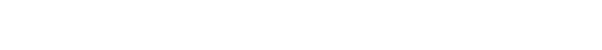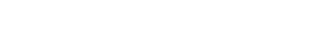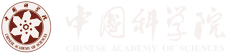Fermionic quantum criticality in fermion-bag inspired Hamiltonianlattice field theories

Date: 2020-01-17
Time: 10:30
Venue: M830
Speaker: Emilie Huffman

Perimeter Institute

Abstract: Motivated by the fermion bag approach--a quantum Monte Carlo approach that takes advantage of grouped local degrees of freedom--we construct a new class of Hamiltonian lattice field theories that can help us study fermionic quantum critical points. We construct the partition function of a lattice Hamiltonian in $2+1$ dimensions in discrete time, with a temporal lattice spacing $\varepsilon$. When $\varepsilon \rightarrow 0$, we obtain the partition function of the original lattice Hamiltonian. But when $\varepsilon = 1$, we obtain a new type of space-time lattice field theory which treats space and time differently, but still lacks fermion doubling in the time dimension, in contrast to Lagrangian lattice field theories. Here we show that both continuous-time and discrete-time lattice field theories derived from the $t$-$V$ model have a fermionic quantum critical point with critical exponents that match within errors. The fermion bag algorithms run relatively faster on the discrete-time model and allow us to compute quantities even on $100^3$ lattices near the quantum critical point. We then discuss how this class of fermion-bag amenable Hamiltonian lattice field theories also include simple $Z_2$ gauge theories coupled to fermions, and explore one such theory in $1+1$ dimensions with a coupling $h$. We demonstrate how even in such a simple theory nontrivial behavior arises, from the emergence of the Gauss law at low temperature to a nontrivial mass scaling.

Contact: Zi Yang Meng (82649331)